# Discover the beauty of algorithms-ranking

Posted Jun 5, 202013 min read• What is sorting?

• First acquaintance
• Algorithm diagram
• Sorting in JavaScript

• Ordinary sorting
• Complex sorting
• Complex sorting function package
• lodash(v4.17.15) sorting function
• See sort() from V8 source code
• The classic sorting algorithm

• Bubble sorting(maximum sorting order)
• Select sorting(minimum value sorting)
• Insert sorting(look for position sorting)
• Merge sorting(binary recursive sorting)
• Quick sorting(basic recursive sorting)
• Leetcode sorting solution problem

1. Search the insertion position(easy)
1. Merge two ordered arrays(easy)
1. The number of bits 1(easy)
1. The shortest unordered continuous sub-array(easy)
1. Array number conversion(easy)
1. Merging interval(medium)
1. The Kth largest element in the array(medium)
• Reference materials

### What is sorting?

#### First acquaintance

There are also many sorts in life, such as descending order of the total score after the exam:
700 points in the first place, 699 points in the second place, 698 points in the third place, etc.
It is worth noting that although the scores are in reverse order, the ranking is in positive order 1, 2, 3...

There are too many examples of sorting in life, so I will not repeat them one by one.

• The sorting English name is sort
• Sorting is a process that turns an unordered(random) set of data into an order
• Ordering is usually divided into two types:ascending(asc) and descending(desc)
• Sorting is very common in software development:front-end data sorting, back-end database lookup table ascending(asc), descending(desc)
• Many algorithms rely on sorting algorithms:stack algorithm, binary search, etc.

#### Algorithm diagram

##### disorder##### descending order##### Ascending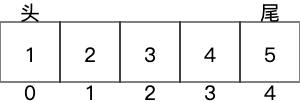### Sorting in JavaScript

In js, the sort() function on Array.prototype can easily meet our requirements for ascending and descending order.

• sort() can be ascending or descending
• After sort() sorting, the array itself changes, no new array is generated

#### Ordinary sort

Suppose you want to sort [2,4,3,1,5]:

``````const arr = [2,4,3,1,5]
//ascending
arr.sort((a,b)=>a-b)
//descending order
arr.sort((a,b)=>b-a)``````

#### Complex sorting

For complex situations, for example, you need to sort the object array according to a key in the object.

``````//items are sorted by value
const items = [
{name:'Edward', value:21 },
{name:'Sharpe', value:37 },
{name:'And', value:45 },
{name:'The', value:-12 },
{name:'Magnetic', value:13 },
{name:'Zeros', value:37}``````

];
items.sort((a, b) => a.value-b.value);

#### Complex sorting function package

``````//key need to sort the key
//Ascending or descending
function sortBy(arr, key, type ='asc'){
if(!arr || !key) return;
let callback =(a, b) => a[key]- b[key]:
if(type ==='desc'){
callback =(a, b) => b[key]- a[key];
}
arr.sort(callback);
}``````

#### lodash(v4.17.15) sorting function

• _.sortedIndex(array, value)
• _.sortedIndexBy(array, value, [iteratee=_.identity])
• _.sortedIndexOf(array, value)
• _.sortedUniq(array)
• _.sortedUniqBy(array, [iteratee])

_.sortBy(collection, [iteratees=[_.identity]])

##### Insert position
``_.sortedIndex([30, 50], 40); //=> 1``
##### Complex insertion position
``````var objects = = [{'''s:4'}, {{''s':5]}];
A
_.sortedIndexBy(objects, {{''x':4}}, function(o){{return}o.x;}});
//==0
A
//The ``..property`iteratee shorthand.
_.sortedIndexBy(objects, {{'x':4}},'x');
//==0``````
##### Query the first index
``_.sortedIndexOf([4, 5, 5, 5, 6], 5); //=> 1``
##### Deduplication and sorting
``_.sortedUniq([1, 1, 2]); //=> [1, 2]``
##### Complex deduplication and sorting
``_.sortedUniqBy([1.1, 1.2, 2.3, 2.4], Math.floor);//=> [1.1, 2.3]``
##### Sort by a key
``````var users = [
{'user':'fred','age':48 },
{'user':'barney','age':36 },
{'user':'fred','age':40 },
{'user':'barney','age':34}``````

];

``````_.sortBy(users, [function(o) {return o.user; }]);
//=> objects for [['barney', 36], ['barney', 34], ['fred', 48], ['fred', 40]]

_.sortBy(users, ['user','age']);
//=> objects for [['barney', 34], ['barney', 36], ['fred', 40], ['fred', 48]]``````

#### See sort() from V8 source code

• The sorting function inside the V8 source code is called InnerArraySort

• The InnerArraySort sorting algorithm is not just a sorting algorithm with multiple optimizations

• InnerArraySort sorting algorithm is integrated into quick sorting and insert sorting

• For arrays with array length less than 22, use insert sort

• For arrays with array length greater than or equal to 22, use quick sort

function InnerArraySort(array, length, comparefn) {
//In-place QuickSort algorithm.
//For short(length <= 22) arrays, insertion sort is used for efficiency.
var InsertionSort = function InsertionSort(a, from, to) {
for(var i = from + 1; i <to; i++) {

``````var element = a[i];
for(var j = i-1; j >= from; j--) {
var tmp = a[j];
var order = comparefn(tmp, element);
if(order> 0) {
a[j + 1]= tmp;
} else {
break;
}
}
a[j + 1]= element;``````

}
};

var QuickSort = function QuickSort(a, from, to) {
var third_index = 0;
while(true) {

``````//Insertion sort is faster for short arrays.
if(to-from <= 10) {
InsertionSort(a, from, to);
return;
}
if(to-from> 1000) {
third_index = GetThirdIndex(a, from, to);
} else {
third_index = from +((to-from) >> 1);
}
//Find a pivot as the median of first, last and middle element.
var v0 = a[from];
var v1 = a[to-1];
var v2 = a[third_index];
var c01 = comparefn(v0, v1);
if(c01> 0) {
//v1 <v0, so swap them.
var tmp = v0;
v0 = v1;
v1 = tmp;
} //v0 <= v1.
var c02 = comparefn(v0, v2);
if(c02 >= 0) {
//v2 <= v0 <= v1.
var tmp = v0;
v0 = v2;
v2 = v1;
v1 = tmp;
} else {
//v0 <= v1 && v0 <v2
var c12 = comparefn(v1, v2);
if(c12> 0) {
//v0 <= v2 <v1
var tmp = v1;
v1 = v2;
v2 = tmp;
}
}
//v0 <= v1 <= v2
a[from]= v0;
a[to-1]= v2;
var pivot = v1;
var low_end = from + 1; //Upper bound of elements lower than pivot.
var high_start = to-1; //Lower bound of elements greater than pivot.
a[third_index]= a[low_end];
a[low_end]= pivot;

//From low_end to i are elements equal to pivot.
//From i to high_start are elements that haven't been compared yet.
partition:for(var i = low_end + 1; i <high_start; i++) {
var element = a[i];
var order = comparefn(element, pivot);
if(order <0) {
a[i]= a[low_end];
a[low_end]= element;
low_end++;
} else if(order> 0) {
do {
high_start--;
if(high_start == i) break partition;
var top_elem = a[high_start];
order = comparefn(top_elem, pivot);
} while(order> 0);
a[i]= a[high_start];
a[high_start]= element;
if(order <0) {
element = a[i];
a[i]= a[low_end];
a[low_end]= element;
low_end++;
}
}
}
if(to-high_start <low_end-from) {
QuickSort(a, high_start, to);
to = low_end;
} else {
QuickSort(a, from, low_end);
from = high_start;
}``````

}
};

if(length <2) return array;
QuickSort(array, 0, num_non_undefined);
return array;
}

### The classic sorting algorithmThere are ten(several) classic sorting algorithms. Due to the current limited capabilities, I will first introduce five sorting algorithms:`bubble, select, insert, merge and fast sort`.

After reading the V8 source code of the sort() function, do you feel that it is "wow so difficult"? In addition to being in awe, in fact, I understand that the algorithm will be constantly optimized. The sort() function has processed a lot according to the characteristics of the JavaScript language. Performance optimization.
Generally speaking, we are happy to use the sort() function that is easy to use and convenient, but there are some classic sorting algorithms, which are still worth exploring.
Even if I have studied in the data structure class, in fact, after a long time, too many bricks are moved, it is still easy to forget. Even if I have not forgotten, I will look back at the algorithm after a few years of work, and may make an optimization for the past algorithm.

The 912 question of LeetCode is a good oj environment, suitable for verifying your own sorting algorithm, recommended to everyone.

• Bubble sorting(maximum sorting order)
• Select sorting(minimum value sorting)
• Insert sorting(look for position sorting)
• Merge sorting(binary recursive sorting)
• Quick sorting(basic recursive sorting)

#### Bubble sorting(maximum sorting at the end)

``````  /**
* Solution:Bubble sort
* Idea:The outer layer keeps putting the maximum value at the end of each cycle, and the minimum value pops forward like a bubble
* Performance:4704ms 39.4MB
* Time complexity:O(n^2)
*/
var sortArray = function(nums) {
for(let i = 0; i <nums.length; i++) {
for(let j = 0; j <nums.length-1-i; j++) {
if(nums[j]> nums[j + 1]) {
const temp = nums[j];
nums[j]= nums[j + 1];
nums[j + 1]= temp;
}
}
}
return nums;
}``````#### Selection sorting(minimum value sorting)

``````  /**
* Solution:select sort
* Idea:sorted interval and unsorted interval. Find the smallest number in the unsorted interval, exchange with the first item of the unsorted interval(the next item in the sorted interval), construct the sorted interval from []to [...], and finally complete the sorting. If it is in descending order, find the largest number.
* Performance:2104ms 41.5MB
* Time complexity:O(n^2)
*/
var sortArray = function(nums) {
for(let i = 0; i <nums.length; i++) {
let min = nums[i];
let idx = i;
for(let j = i + 1; j <nums.length; j++) {
if(nums[j]<min) {
min = nums[j];
idx = j;
}
if(j === nums.length-1) {
let temp = nums[i];
nums[i]= nums[idx];
nums[idx]= temp;
}
}
}
return nums;
}``````#### Insert sorting(look for position sorting)

``````  /**
* Solution:Insert sort
* Idea:sorted interval and unsorted interval. Take the first item of the unsorted range, find your position on the sorted range, generally find foo<x<bar,   insert x between foo and bar, or insert x<bar into the head.
* Key point:stop searching in the sorted array immediately after inserting into the specified position
* Performance:2008ms 43.9MB
* Time complexity:O(n^2)
* */
var sortArray = function(nums) {
const sorted = [nums];
for(let i = 1; i <nums.length; i++) {
//j = i-1; also works
for(let j = sorted.length-1; j >= 0; j--) {
if(nums[i]<sorted[j]) {
if(j === 0) {
sorted.splice(j, 0, nums[i]);
}
} else if(nums[i]>= sorted[j]) {
sorted.splice(j + 1, 0, nums[i]);
j = -1; //this is very important, stop searching in the sorted array immediately after inserting into the specified position
}
}
}
return sorted;
}
/**
* Optimized version:Insert sort(without the help of auxiliary array)
* Idea:insert splice(j/j+1, 0), delete splice(i, 1)
* It should be noted that splice() returns an array, for example 
* Performance:2372ms 42.5MB
* Time complexity:O(n^2)
*/
var sortArray = function(nums) {
for(let i = 1; i <nums.length; i++) {
for(let j = i-1; j >= 0; j--) {
if(nums[i]<nums[j]) {
if(j === 0) {
nums.splice(j, 0, nums.splice(i, 1));
}
} else if(nums[i]>= nums[j]) {
nums.splice(j + 1, 0, nums.splice(i, 1));
j = -1;
}
}
}
return nums;
}``````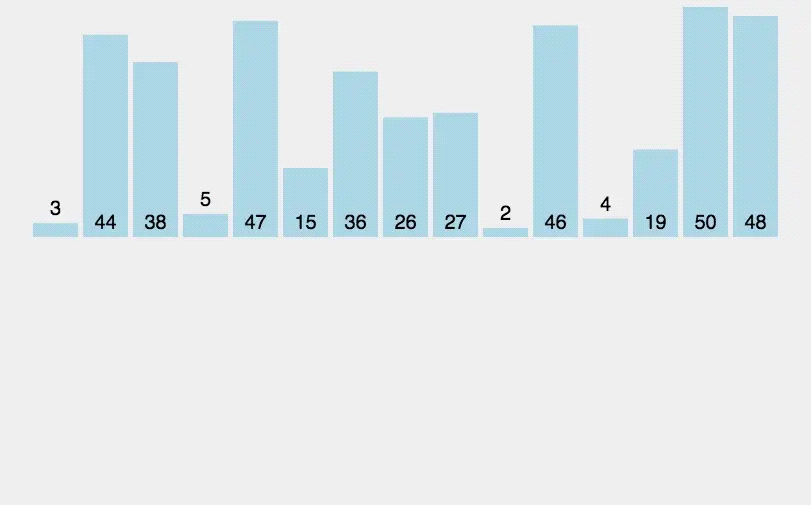#### Merging and sorting(binary recursive sorting)

``````  /**
* Solution:merge sort
* Idea:Split an array of length n into an array of length n/2, and sort them separately. Then merge the n/2 length arrays until the final sorted array length is 2, and finally merge the final sorted arrays up one after the other
* Core:dichotomy and recursion. Similar to binary sorting, the sorting of top-down dichotomous disassembling and bottom-up merging of sorting results.
* Note:The condition for terminating recursion is if(length <= 1) {return nums;}
* Performance:260ms 47.9MB
* Time complexity:O(nlogn)
*/

var sortArray = function(nums) {
const merge =(left, right) => {
const result = [];
while(left.length && right.length) {
if(left>= right) {
result.push(right.shift());
} else {
result.push(left.shift());
}
}
while(left.length) {
result.push(left.shift());
}
while(right.length) {
result.push(right.shift());
}
return result;
};
let length = nums.length;
if(length <= 1) {
return nums;
}
let middle = Math.floor(length/2);
let left = nums.splice(0, middle);
let right = nums;
return merge(sortArray(left), sortArray(right));
}``````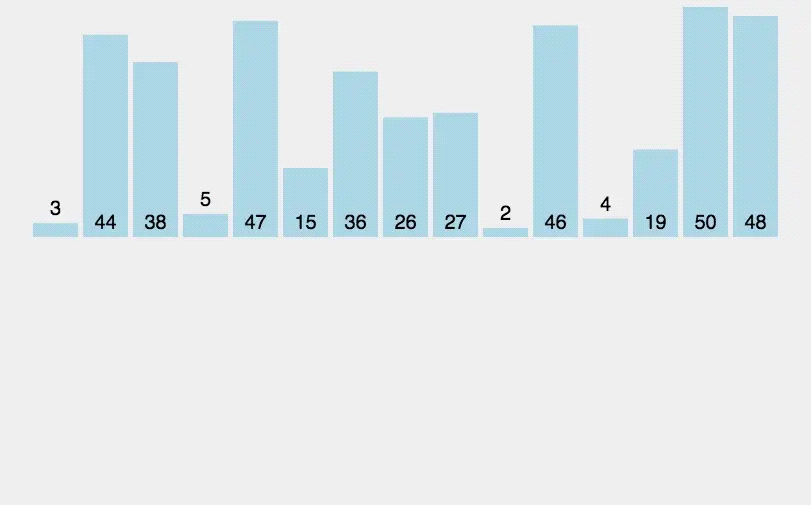#### Quick sorting(basic recursive sorting)

``````  /**Solution:Quick Sort
* Ideas:
* 1. Select a split point split
* 2. Define the left and right double pointers, and place the smaller division value on the left side and the larger division value on the right side in one traversal
* 2.1 swap(left, right) when the left and right pointers do not meet
* 2.2 When left and right pointers meet, swap(start, left) and return left
* 3. The divide-and-conquer recursion is a sequence of left and right sides***
* Performance:128ms 40.8MB
* Time complexity:O(nlogn)
*/
var sortArray = function(nums) {
quickSort(nums, 0, nums.length-1);
return nums;
//define a *** function
function quickSort(arr, left, right) {
if(left <right) {
let splitIndex = findSplitIndex(nums, left, right);
quickSort(nums, left, splitIndex-1);
quickSort(nums, splitIndex + 1, right);
}
}
//Find the split value index
function findSplitIndex(arr, left, right) {
const start = left;
const splitValue = arr[start];
while(left !== right) {
while(left <right && arr[right]> splitValue) {
right--;
}
while(left <right && arr[left]<= splitValue) {
left++;
}
if(left <right) {
swap(arr, left, right);
}
}
swap(arr, start, left);
return left;
}
//Exchange position:left and right exchange, split point and left exchange
function swap(arr, i, j) {
const temp = arr[j];
arr[j]= arr[i];
arr[i]= temp;
}
};``````

Algorithm process diagram(from the programmer's article:[Comic:What is a quick sort?(full version)]( https://mp.weixin.qq.com/s?__biz=MzIxMjE5MTE1Nw%3D%3D&chksm=8c99f9f8bbee70eef627d0f5e5b80a604221abb3a1b56bbbbbfbxc 1&mid=2653195042&scene=21&sn=2b0915cd2298be9f2163cc90a3d464da#wechat_redirect))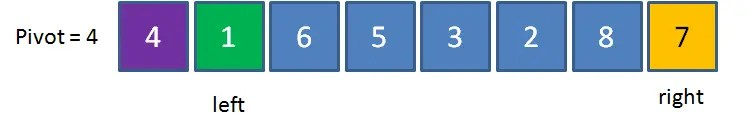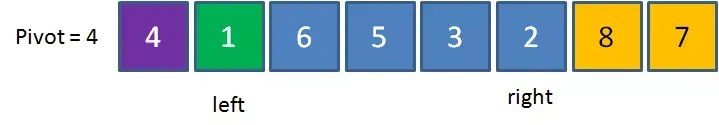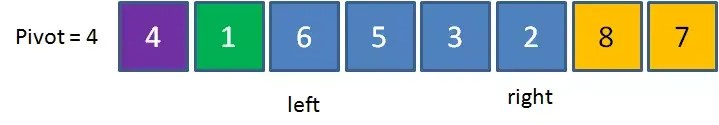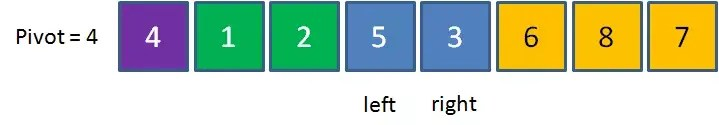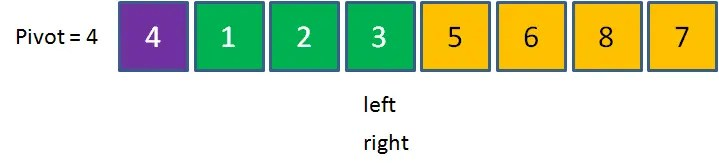### leetcode sort solution problem

1. Search the insertion position(easy)
1. Merge two ordered arrays(easy)
1. The number of bits 1(easy)
1. The shortest unordered continuous sub-array(easy)
1. Array number conversion(easy)
1. Merging interval(medium)
1. The Kth largest element in the array(medium)

#### 35. Search for insertion location

``````var searchInsert = function(nums, target) {
/**
* Solution 2:Push array reordering method 96ms better than 6.35%
*/
nums.push(target);
var resortedNums = nums.sort((x,y)=>x-y);
return resortedNums.indexOf(target);
};``````

#### 88. Merge two ordered arrays(easy)

``````var merge = function(nums1, m, nums2, n) {
/**
* Special points to note:This question will check the final storage of the nums1 array memory space
*/
//splice truncates the array
nums1.splice(m);
nums2.splice(n);
//Reason for not using concat:concat returns a new array, and the title needs to be stored directly in the space of nums1
nums2.forEach(num2 => {
nums1.push(num2);
});
//sort sorts the current array
var ascArr = nums1.sort((a, b) => a-b);
return ascArr;
};``````

#### 191. The number of bit 1(easy)

``````/**
* @param {number} n-a positive integer
* @return {number}
*/
var hammingWeight = function(n) {
/**Solution 4:Sort optimization count
* Performance:88ms 35.7MB
*/
let strArr = n.toString(2).split("");
strArr.sort((a, b) => parseInt(b)-parseInt(a));
let count = 0;
for(let i = 0; i <strArr.length; i++) {
if(strArr[i]=== "1") count++;
}
return count;
};``````

#### 581. The shortest unordered continuous sub-array(easy)

``````/**
* @param {number[]} nums
* @return {number}
*/
var findUnsortedSubarray = function(nums) {
/**
* Solution
*-Clone array and sort
*-Find the index value of the starting element
*-startIdx finds the index of the first changed element from beginning to end
*-endIdx finds the index of the first changed element from end to end
*/
//Use [...nums]to clone a new array, because sort changes itself and does not return a new array
var sortedNums = [...nums].sort((a, b) => a-b);
var startIdx = 0;
for(var i = 0; i <nums.length; i++) {
if(nums[i]!== sortedNums[i]) {
startIdx = i;
break;
}
}
var endIdx = 0;

for(var j = nums.length-1; j >= 0; j--) {
if(nums[j]!== sortedNums[j]) {
endIdx = j;
break;
}
}
var length = endIdx-startIdx> 0? endIdx-startIdx + 1:0;
return length;
};``````

#### 1331. Array number conversion(easy)

``````/**
* @param {number[]} arr
* @return {number[]}
*/
var arrayRankTransform = function(arr) {
if(arr.length> Math.pow(10, 5)) return;
/**
* Generate unique sorted Map
*/
var uniqArr = Array.from(new Set(arr));
var sortArr = uniqArr.sort((a, b) => a-b);
//Construct a two-dimensional array as a parameter of the Map constructor
var twoDimArr = sortArr.map((num, idx) => [num, idx + 1]);
var idxMap = new Map(twoDimArr);
/**
* Find number serial number in Map
*/
var serialNums = [];
for(var i = 0; i <arr.length; i++) {
serialNums.push(idxMap.get(arr[i]));
}
return serialNums;
};``````

#### 56. Merging interval(medium)

``````/**
* @param {number[][]} intervals
* @return {number[][]}
*/
var merge = function(intervals) {
/**
* Solution 1:Sort + Stack
* Performance:88ms 36.3MB
* Ideas:
* Push into the interval empty stack or arris greater than the last interval of the stack closed
* Overlap overlap arris less than the last interval of the stack
* */
intervals.sort((a, b) => a-b);
let stack = [];
for(let i = 0; i <intervals.length; i++) {
let currrentInterval = intervals[i];
let stackLastItem = stack[stack.length-1];
if(stack.length === 0 || currrentInterval> stackLastItem) {
stack.push(currrentInterval);
} else if(currrentInterval<= stackLastItem) {
stackLastItem= Math.max(stackLastItem, currrentInterval);
}
}
return stack;
};``````

#### 215. The Kth largest element in the array(medium)

``````var findKthLargest = function(nums, k) {
/**
* Solution 1:Output in reverse order
*/
nums.sort((a, b) => b-a);
return nums[k-1];
};``````

Looking forward to communicating with you and making progress together, welcome everyone to join the technical discussion group I created that is closely related to front-end developmentStrive to become an excellent front-end engineer!